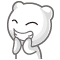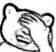# 博客

## 清华集训总结

2015-12-11 14:08:29 By matthew99

## 分金币问题

2015-10-26 15:56:32 By matthew99

## app管理器的乱搞经历

2015-08-11 09:06:46 By matthew99

## ...妈妈我终于会做动态仙人掌IV了

2015-05-16 23:10:45 By matthew99UPD:出了点奇怪的事情。

## mx的仙人掌 题解

2015-03-27 11:59:14 By matthew99

#### 题目地址

http://uoj.ac/problem/87

#### 算法二

##### 更好的做法

$\mathrm{dfs}$完以后，对每个点考虑他到根的距离，如果他没有环标记那么距离就是父亲到根的距离加上自己到父亲的边的长度。否则就是他到环的根$y$的两条路径长度(一个是$\mathrm{dfs}$树上的距离(这个用两个点离根的距离减一减就求出来了)，另一个是环的长度减去树上距离)中的较小值加上$y$到根的距离。

#### 算法五

UPD:树上迭代两次求最远点即可，最远点可以直接枚举然后$\mathrm{lca}$，呜呜呜我出题的时候怎么就脑抽了，30分送大家吧，前面的算法就当做口胡......

#### 算法六

UPD:好像可以记录到根的距离，然后用特殊的方法处理一下就可以直接减了。总之实现上有各种技巧优化时间复杂度和代码复杂度，感觉标程就是个逗比啊TAT......

#### 解法八

AC代码：

http://uoj.ac/submission/13387

http://uoj.ac/submission/13388

#### 解法九

waltz给出了另外一种解法：http://uoj.ac/submission/13649

#### 后记

5s的时限真是太良心了233，主要是为了欢迎各种奇葩做法。

## mx的组合数 题解

2015-03-27 11:58:56 By matthew99

#### 题目地址

http://uoj.ac/problem/86

QAQ我把这题出出来之后，发现以前有一道几乎一样的题，而且我还A过！感觉自己记性真的有点好......#### 算法三

${a \choose b} \bmod p = {\lfloor\frac{a}{p}\rfloor \choose \lfloor\frac{b}{p}\rfloor}{a \bmod p \choose b \bmod p} \bmod p$

${a \choose b} \bmod p = \prod\limits_{i \ge 0}{a_i \choose b_i} \bmod p$，其中$a_i$表示$a$在$p$进制下从较低位数起的第$i$位。

#### 算法四

$g[i, j]$表示从低位开始前$i$位任意且值为$j$的方案数。 $f[i, j]$表示从低位开始前$i$位对应的$p$进制数不超过$bound$的前$i$位对应的$p$进制数且值为$j$的方案数。

$g[i + 1, jz \bmod p]$ += $g[i, j]$

#### 算法五## π(n)的计算

2015-03-11 09:23:26 By matthew99

$\pi(n)\ne\pi n$，更不是$3.141592655\cdots (n)$(←_←)，而是小于等于$n$的质数个数，$n$可以是任意实数。

#### 帅气的算法一

$\lfloor x\rfloor - \sum\limits_{i}\lfloor\frac{x}{p_i}\rfloor + \sum\limits_{i\lt j}\lfloor\frac{x}{p_ip_j}\rfloor - \sum\limits_{i\lt j\lt k}\lfloor\frac{x}{p_ip_jp_k}\rfloor + \cdots$

$\Phi(x', n') = \Phi(x', n' - 1) - \Phi(\frac{x'}{p_{n'}}, n' - 1)$

$\Phi(x', 0) = \lfloor x'\rfloor$

UPD：任之洲同学指出这个算法的计算量实际上是$O(\frac{x}{\log x})$，且给出了一个$O(\frac{n ^ \frac{3}{4}}{\log n})$的做法，并且可以推广到质数的$k$次方和的情况。看起来本文没有什么存在的意义了(雾)。

#### 帅气的算法二

$\Phi(x, n) = \sum\limits_{k = 0}^{+∞}P_k(x, n)$

$P_1(x, n) = \pi(x) - n$

$P_2(x, n) = \sum\limits_{y \lt p \le \sqrt x, p是质数}{\pi(\frac{m}{p}) - \pi(p) + 1}$(设$x = ab$($a, b$是质数)是满足条件的数，那么当且仅当$p = min\{a, b\}$时统计了$x$一次)

$\pi(x) = \Phi(x, n) - 1 + n - (\sum\limits_{y \lt p \le \sqrt x}{\pi(\frac{m}{p}) - \pi(p) + 1})$#### 实现

#include <cstdio>
#include <cstdlib>
#include <cstring>
#include <algorithm>
#include <cmath>
#include <cctype>
#include <ctime>
#include <cassert>
#include <ext/pb_ds/assoc_container.hpp>
#include <iostream>

#define REP(i, a, b) for (int i = (a), _end_ = (b); i != _end_; ++i)
#define debug(...) fprintf(stderr, __VA_ARGS__)

using namespace std;

typedef long long LL;

const int maxn = 1e6, max0 = 1e5;

int sum[maxn + 5];
int prime[max0 + 5];
int pn = 0;

LL n = 1000000000LL;

int Max = -1;

__gnu_pbds::gp_hash_table<int, int> id;

LL a;
int an = 0;

LL calc(const int &n, const int &x)
{
if (x < 0) return n;
if (!id[n]) id[n] = ++an;
int tmp = id[n];
if (a[tmp][x] != -1) return a[tmp][x];
return a[tmp][x] = calc(n, x - 1) - calc(n / prime[x], x - 1);
}

int main()
{
memset(a, -1, sizeof a);
for (int i = 2; i <= maxn; ++i)
{
if (!sum[i])
{
if ((LL)i * i * i <= n) Max = pn;
prime[pn++] = i;
}
sum[i] = sum[i - 1] + !sum[i];
for (int j = 0; j < pn && prime[j] * i <= maxn; ++j)
{
sum[i * prime[j]] = 1;
if (!(i % prime[j])) break;
}
}
LL ans = calc(n, Max) + Max;
for (int p = Max + 1; (LL)prime[p] * prime[p] <= n; ++p) ans -= sum[n / prime[p]] - sum[prime[p]] + 1;
cout << ans << endl;
return 0;
}

#### 参(chao)考(xi)文献

Prime-counting function

UPD:本文章中的算法已被完艹...更多内容参见π(n)的计算++

## ...妈妈我终于会玩新年的魔塔了！！

2015-03-06 01:20:09 By matthew99## 已未年第一篇博客，祝大家新年快乐

2015-02-19 00:04:28 By matthew99